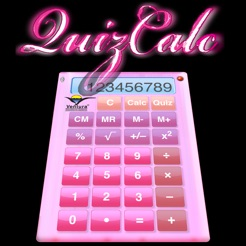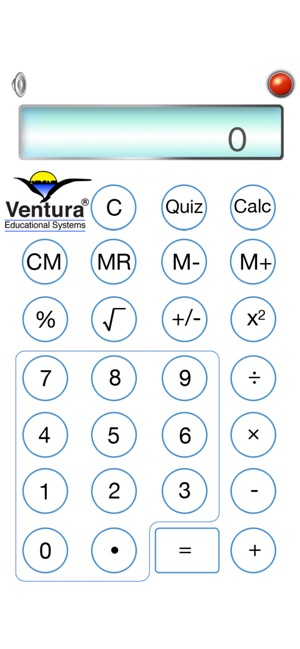Screenshots

•Description

QuizCalc is a basic calculator perfect for elementary age students with percent, square root, integer, power and memory ( +, – , C & R ) functions. Tap the Quiz button and QuizCalc becomes a math quiz generator providing students with a great way to practice math facts and concepts.

Here are a few of the ways you will use QuizCalc everyday with your elementary age students:

• Use QuizCalc just like any other calculator to help with math studies.

•Tap the Quiz button and QuizCalc shifts into a game where students practice arithmetic facts.

•Use QuizCalc everyday to improve scores on 8 different randomly generated quizzes at 2 levels.

In addition to functioning as a regular calculator, QuizCalc provides students with practice in the following mental math areas:

Math Objectives
•Addition Facts with Numbers from 0 to 10.
•Addition Facts with Numbers from 10 to 40.
Subtraction of Whole Numbers
•Subtraction Facts with Numbers from 0 to 20
•Subtraction Facts with Numbers from 10 to 60
Multiplication of Whole Numbers
•Multiplication Facts with Numbers from 0 to 10
•Multiplication Facts with Numbers from 0 to 20
Division of Whole Numbers
•Division Facts with Divisors from 1 to 10.
•Division Facts with Divisors from 1 to 20.
Percents
•Problems with Percents from 10 to 100.
•Problems with Percents from 10 to 200.
Roots of Perfect Squares
•Find Roots from 1 to 10.
•Find Roots from 5 to 12.
•Numbers from -5 to +5.
•Numbers from -10 to +10.
Perfect Squares
•Find the Square of Numbers from 1 to 10.
•Find the Square of Numbers from -10 to 10.

Tap the Calculator button to switch QuizCalc into Calculator Mode. In Calculator Mode, QuizCalc works just like any other algebraic calculator. Here are some examples of how to do some basic calculations:

Operation Keystrokes Display
addition 1 + 2 = 3
subtraction 10 - 7 = 3
multiplication 5 × 8 = 40
division 60 ÷ 12 = 5
percent 100 + 10 % = 110
square root 81 √ 9
integer 12 +/- -12

What’s New

Version 3.0

This is a regular maintenance update.

Ratings and Reviews

5.0 out of 5
1 Rating

1 Rating

iPhoneAppRevieweMan ,

Simple and works well

Like the skin options. The quiz is simple and easy for a child to use...fun! Worth the price.

Information

Seller
Ventura Educational Systems
Size
29.3 MB
Category
Education
Compatibility

Requires iOS 8.0 or later. Compatible with iPhone, iPad, and iPod touch. Apple TV.

Languages

English

Age Rating
Rated 4+
•